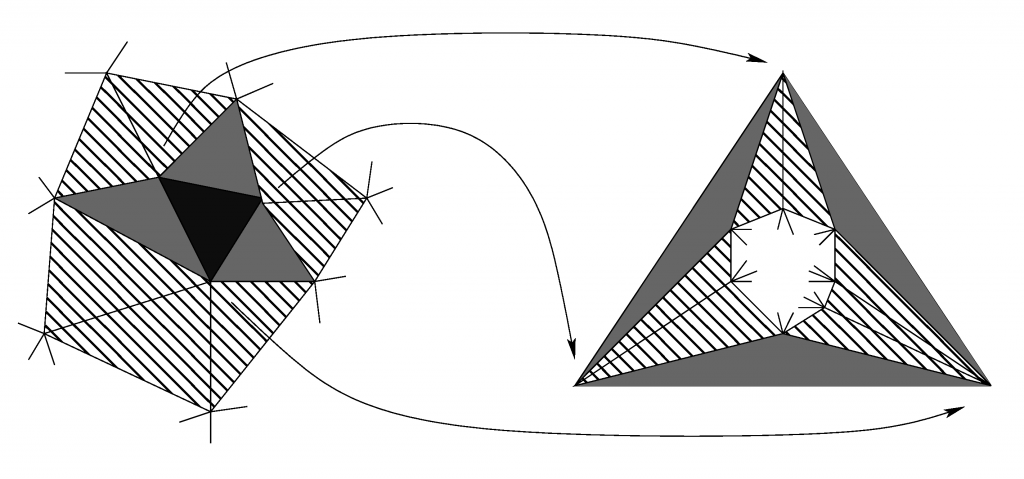# Stable broken H^1 and H(div) polynomial extensionsMapping of the boundary of a 3D tetrahedral patch to a triangulation for three-color patch refinement

Main results:

• H^1-stable polynomial extension on a tetrahedron;
• H(div)-stable polynomial extension on a tetrahedron;
• stable broken H^1 polynomial extension on a patch of thetrahedra;
• stable broken H(div) polynomial extension on a patch of thetrahedra;
• polynomial-degree-robust efficiency of H^1-nonconforming methods;
• polynomial-degree-robust efficiency of H(div)-nonconforming methods.

Details: paper with Alexandre Ern (preprint) and presentation